### Home > CALC > Chapter 10 > Lesson 10.1.8 > Problem10-78

10-78.
1. Examine the following series. Use one of the tests you have learned so far to determine if the series converges or diverges. State the test that you used. Homework Help ✎

1.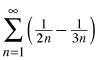2.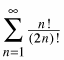3.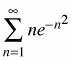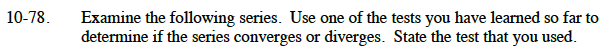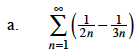$\frac{1}{2n}-\frac{1}{3n}=\frac{1}{6n}$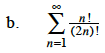$\lim_{n\to\infty}\frac{(n+1)!/(2n+2)!}{n!/(2n)!}$

$=\lim_{n\to\infty}\Bigg(\frac{(n+1)!}{n!}\cdot\frac{(2n)!}{(2n+2)!}\Bigg)$

$=\lim_{n\to\infty}\frac{n+1}{(2n+2)(2n+1)}=?$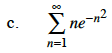$ne^{-n^2}=\frac{n}{e^{n^2}}$

$\lim_{n\to\infty}\frac{(n+1)/e^{(n+1)^2}}{n/e^{n^2}}$